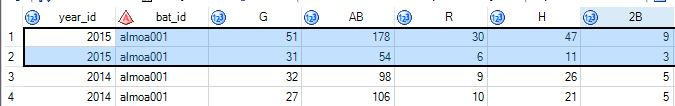Hi,

In my data, I have multiple rows for one person and I'd like to combine all the rows into one unique row while summing the values. For example:Where year_id and bat_id are equal, I would like to combine the rows and sum the column values. So for 2015, I would just want one row with values:

82 for G, 232 for AB, and so one. What procedure is best able to do this, or could it be done in the data step?

Thank you,

Justin

1 ACCEPTED SOLUTION

Accepted Solutions

you could try the proc means

``````data have;
input year_id 	bat_id\$	g	ab	r	h	_2b;
cards;
2015	almoa001	51	178	30	47	9
2015	almoa001	31	54	6	11	3
2014	almoa001	32	98	9	26	5
2014	almoa001	27	106	10	21	5
;

proc means data=have nway;
class year_id bat_id;
var g ab r h _2b;
output out=sums sum=/autoname;
run;
``````

Thanks,
Jag
3 REPLIES 3

you could try the proc means

``````data have;
input year_id 	bat_id\$	g	ab	r	h	_2b;
cards;
2015	almoa001	51	178	30	47	9
2015	almoa001	31	54	6	11	3
2014	almoa001	32	98	9	26	5
2014	almoa001	27	106	10	21	5
;

proc means data=have nway;
class year_id bat_id;
var g ab r h _2b;
output out=sums sum=/autoname;
run;
``````

Thanks,
Jag

alternatively proc sql

``````proc sql;
create table want as select year_id, bat_id,sum(g) as g, sum(ab) as ab, sum(r) as r, sum(h) as h, sum(_2b) as _2b
from have group by year_id,bat_id;
quit;
``````
Thanks,
Jag